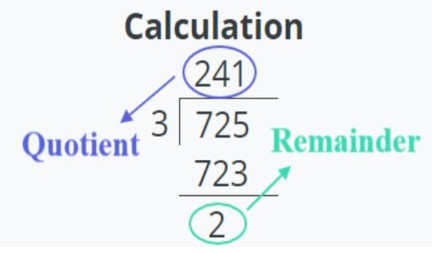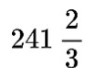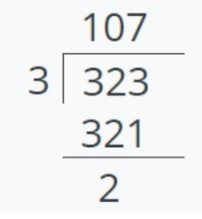# Remainder Calculator

Input the required values i.e dividend and divisor in the division remainder calculator. Press enter to find the result.

Give Us Feedback

Remainder calculator, as the name suggests, is used for finding the left term at the end of a division. This calculator can find three different values for the users. These are:

• Remainder
• Quotient
• Calculation process

## What is a remainder?

The remainder is that whole number that is left or remaining at the end after a division calculation. It happens when the dividend is not completely divisible by the divisor. For instance, see the image below:Such calculations, when put in a fraction, make mixed numbers. The remainder comes as numerator, divisor as the denominator, and the quotient are written at the left side of the fraction.

For example:## How to find the remainder?

You can use the quotient and the remainder calculator to find the remainder. But to learn the hand method, see the example solved below.

Example:

Divide 234 by 3. Also, tell its remainder.

Solution:

Step 1: Identify the values.

Divisor = 3

Dividend = 324

Step 2: Perform division.

= 324 / 3Step 3: Identify the remainder.

The remainder is 2.

Normal scientific calculators do not give remainders to such questions. You usually get a decimal value answer. This is why, remainders calculator is useful.

### Math Tools